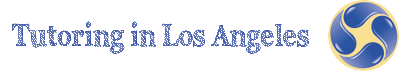# Geometry Tutoring

Geometry is a useful discipline and Tutoring in Los Angeles provides expertise in developing abilities to understand, prove and use geometric theorems, axioms and inductive and deductive reasoning.

Math-using abstract symbols to describe, order, explain, and predict-has become essential to human existence. It is a universal language of numbers that is spoken throughout the world and is as important as oral and written language is in the communication of ideas. Young people who have taken gateway courses such as algebra and geometry go on to college at much higher rates than those who do not. The study of math has contributed to the fields of medicine, management, economics, government, computer science, physics, psychology, engineering, and social science. Math also enables us to conduct the simple yet vital transactions of daily life, such as telling time, gauging distances, and making change.

The geometry skills and concepts developed in this discipline are useful to all students. Aside from learning these skills and concepts, students will develop their ability to construct formal, logical arguments and proofs in geometric settings and problems.

Geometry is the study of space and figures in space. In school any study of space, whether practical or theoretical, is put into the geometry strand. In the early grades this strand includes the use of measuring tools, such as rulers, and recognition of basic shapes, such as triangles, circles, squares, spheres, and cubes. In the later grades the content extends to the study of area and volume and the measurement of angles. In high school, plane geometry is studied both as an introduction to the concept of mathematical proof and as a fascinating structure that has profoundly influenced civilization for more than 2,000 years.

Although the curriculum is weighted heavily in favor of plane (synthetic) Euclidean geometry, there is room for placing special emphasis on coordinated geometry and its transformations.

A principal difficulty with geometry at all levels is the need for precise definitions of geometric concepts. Many students-including high-achieving ones-complete a course in geometry with so little geometric intuition that, given three noncollinear points, they cannot even begin to visualize what the circumcircle of these points must be like. One way to develop this geometric sense is to encourage to draw many pictures to develop a geometric sense and to amass a wealth of geometric data in the process. For example, identifying numbers as points on the real line is an important step in relating students' concepts of arithmetic to geometry. This fusion of arithmetic and geometry, which is ubiquitous in math, adds a new dimension to students' understanding of numbers. All instructional materials used in the tutoring sessions by our tutors not just those for geometry, but especially those for algebra and trigonometry-should carefully present proofs of math assertions when the situation calls for them.

It has become customary in tutoring geometry to start with axioms that incorporate real numbers. Although doing geometric proofs with real numbers runs counter to the spirit of Euclid, this approach is a good math compromise in the context of school math. However, the parallel postulate occupies a special place in geometry and should be clearly stated in the traditional form. An important aspect of teaching three-dimensional geometry is to cultivate students' spatial intuition. Most students find spatial visualization difficult, which is all the more reason to make the teaching of this topic a high priority.

 Phone: (866) 414 2030 Toll Free (310) 405 0721 (818) 850 2030 Fax: (866) 669 9584 Email: tutor@tutoringinla.com